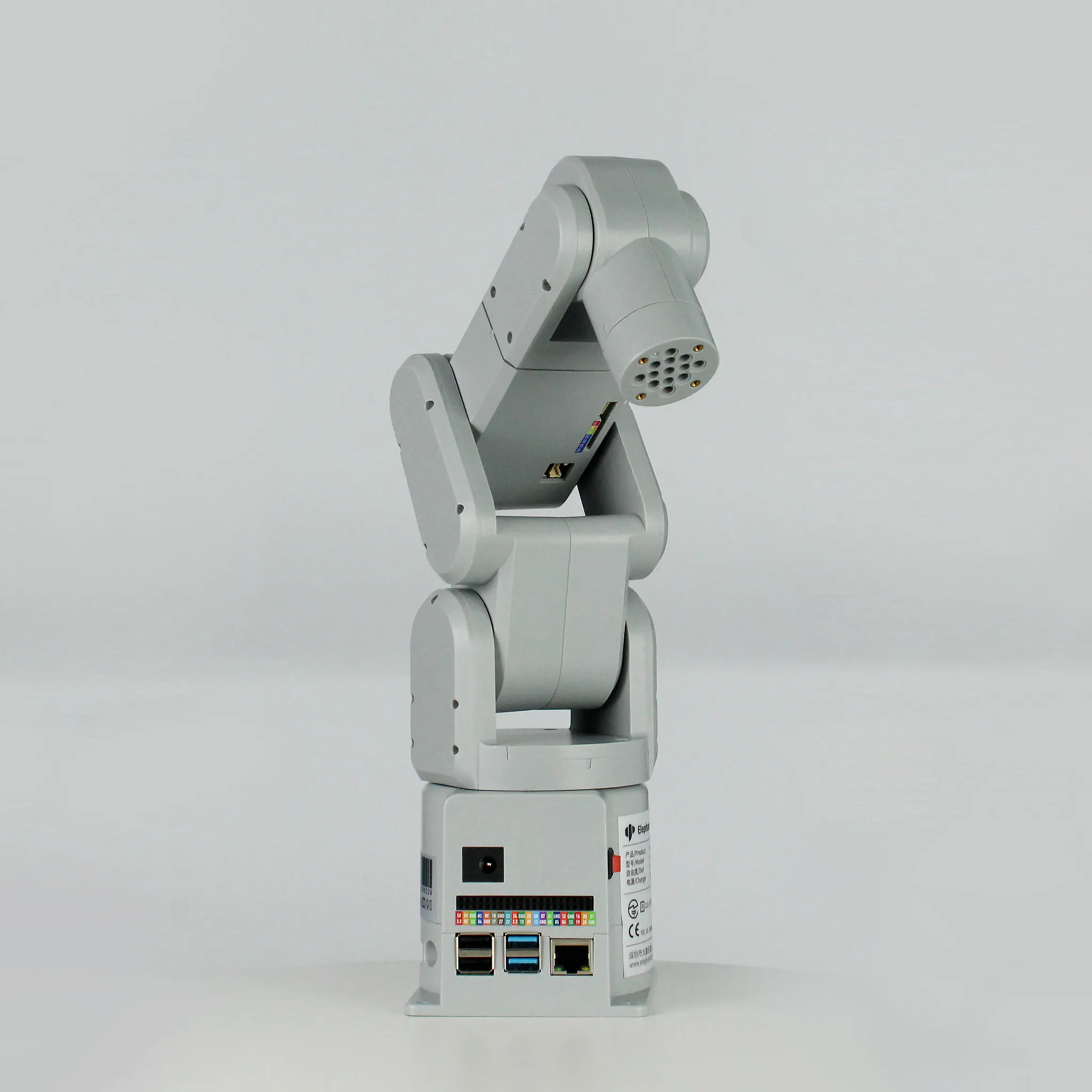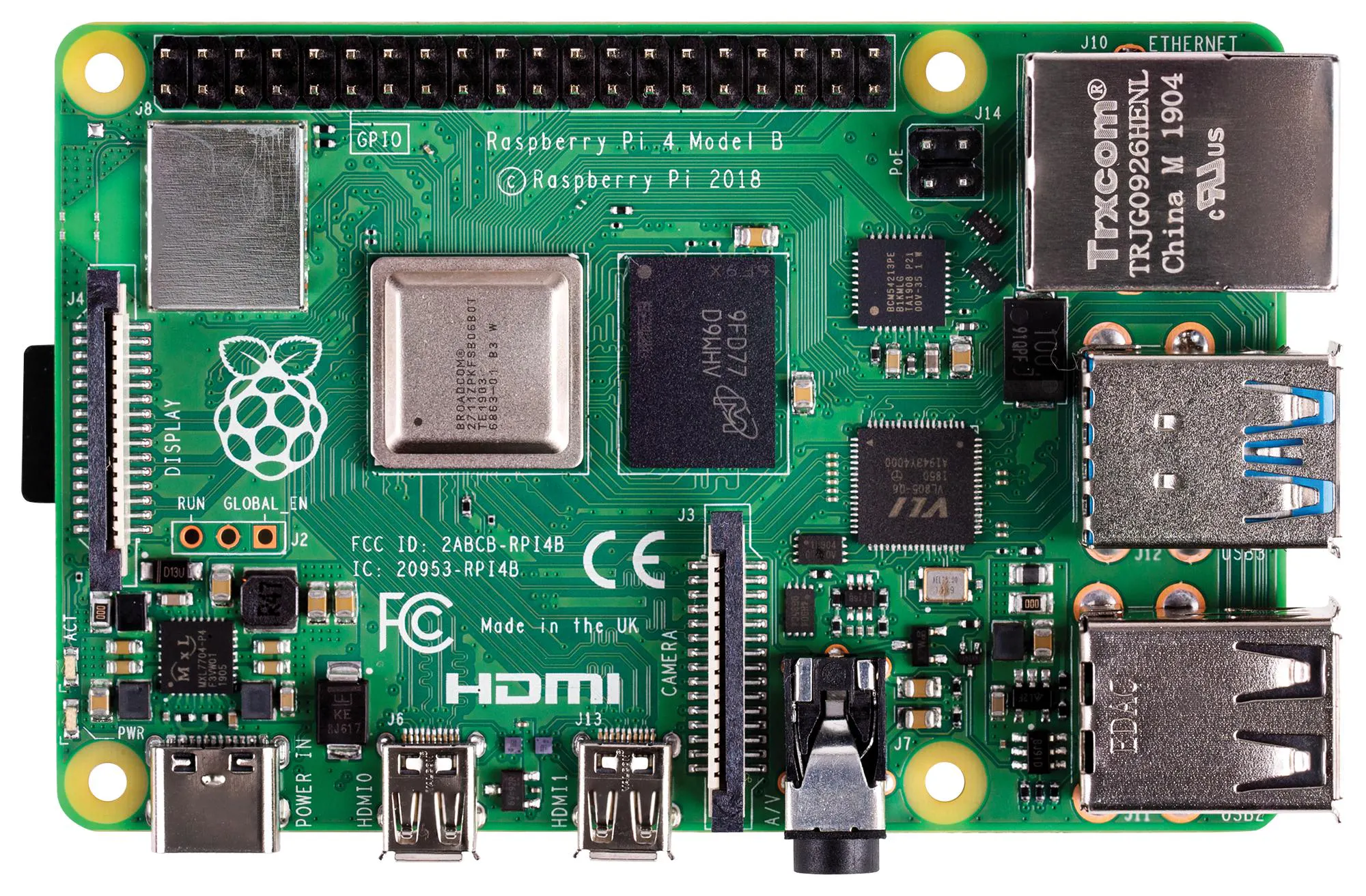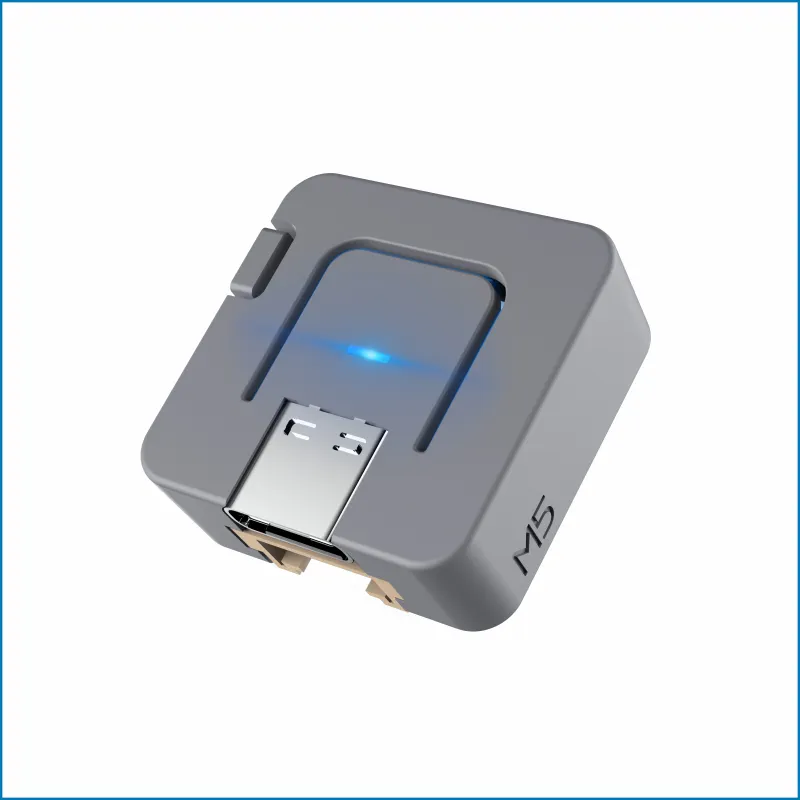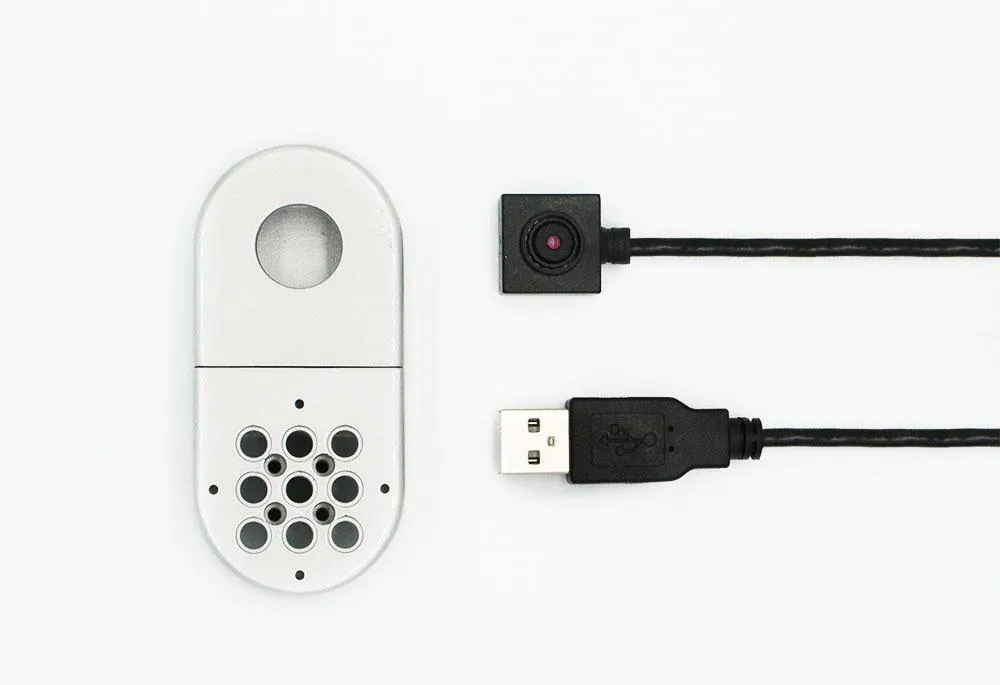Published

# Facial Recognition and Tracking Project with mechArm

IntermediateFull instructions provided2 hours748

## Things used in this project

### Hardware componentsElephant Robotics mechArm 270 Pi
×1Raspberry Pi 4 Model B
×1M5Stack ATOM Lite ESP32 Development Kit
×1

### Software apps and online servicesOpenCV

### Hand tools and fabrication machinesElephant Robotics Camera Flange

## Code

### Code

Python
```from cmath import rect
from pickle import TRUE
import cv2
import math
import matplotlib.pyplot as plt
import time
from pymycobot.mycobot import MyCobot
mc = MyCobot('COM9',115200)

def video_info_fps():

# Input video stream
cap = cv2.VideoCapture(0)
# To use a video file as input
#cap = cv2.VideoCapture('demo.mp4')
'''cal FPS'''
# https://www.geeksforgeeks.org/python-displaying-real-time-fps-at-which-webcam-video-file-is-processed-using-opencv/
# Initialisation flag bit
save_state = False
run_count = 0
# Fixed starting stance
mc.send_angles([0, -23, -5, 0, 28, -90.85], 100)
time.sleep(2)

while True:
# Conversion to greyscale
gray = cv2.cvtColor(img, cv2.COLOR_BGR2GRAY)
# Face detection
# Drawing outlines
for (x, y, w, h) in faces:
if w > 200 or w < 80:   #Limit the recognition width to between 80 and 200 pixels
continue
cv2.rectangle(img, (x, y), (x+w, y+h), (255, 0, 0), 3)
center_x = (x+w-x)//2+x
center_y = (y+h-y)//2+y
size_face = w

# display
cv2.imshow('img', img)
k = cv2.waitKey(30) & 0xff
if k==27:
break
cap.release()

run_num = 20    #Control cycle of 20 frames
if save_state == False:
# Save a start point (save_x, save_y)
save_x = center_x
save_y = center_y
save_z = size_face
origin_angles = mc.get_angles()
print("origin point = ", save_x, save_y, origin_angles)
time.sleep(2);
current_coords = mc.get_coords()
save_state = TRUE
else:
if run_count > run_num: # Limit the control period to 20 frames
run_count = 0
# Record relative offsets
error_x = center_x - save_x
error_y = center_y - save_y
error_z = size_face - save_z

# Pixel differences are converted into actual offsets, which can be scaled and oriented
trace_1 = -error_x * 0.15
trace_z = -error_y * 0.5
trace_x = -error_z * 2.0

# x/z axis offset, note that this is open loop control
current_coords += trace_z
current_coords += trace_x

#Restricting the Cartesian space x\z range
if current_coords < 70:
current_coords = 70

if current_coords > 150:
current_coords = 150

if current_coords < 220:
current_coords = 220

if current_coords > 280:
current_coords = 280

# Inverse kinematic solutions
x = current_coords
z = current_coords
# print(x, z)

L1 = 100;
L3 = 96.5194;
x = x - 56.5;
z = z - 114;

cos_af = (L1*L1 + L3*L3 - (x*x + z*z))/(2*L1*L3);
cos_beta = (L1*L1 - L3*L3 + (x*x + z*z))/(2*L1*math.sqrt((x*x + z*z)));
reset = False
# The solution is only applicable to some poses, so there may be no solution
if abs(cos_af) > 1:
reset = True
if reset == True:
current_coords -= trace_z
current_coords -= trace_x
print("err = ",cos_af)
continue

af = math.acos(cos_af);
beta = math.acos(cos_beta);

theta2 = -(beta + math.atan(z/x) - math.pi/2);
theta3 = math.pi/2 - (af - math.atan(10/96));
theta5 = -theta3 - theta2;
cof = 57.295 #Curvature to angle

move_juge = False
# Limits the distance travelled, where trace_1 joint is in ° and trace_x/z is in mm
if abs(trace_1) > 1 and abs(trace_1) < 15:
move_juge = True
if abs(trace_z) > 10 and abs(trace_z) < 50:
move_juge = True
if abs(trace_x) > 25 and abs(trace_x) < 80:
move_juge = True

if (move_juge == True):
print("trace = ", trace_1, trace_z, trace_x)
origin_angles += trace_1
origin_angles = theta2*cof
origin_angles = theta3*cof
origin_angles = theta5*cof
mc.send_angles(origin_angles, 70)
else:
#Due to the open-loop control, if no displacement occurs the current coordinate value needs to be restored
current_coords -= trace_z
current_coords -= trace_x
else:
# 10 frames set aside for updating the camera coordinates at the end of the motion
if run_count < 10:
save_x = center_x
save_y = center_y
save_z = size_face
run_count += 1
```

## Credits

### i olll

2 projects • 2 followers

### Elephant Robotics

31 projects • 93 followers
An Innovative Robotics Company.